Chapter Notes - Lines and Angles

# Chapter Notes - Lines and Angles Notes | Study Mathematics (Maths) Class 7 - Class 7

## Document Description: Chapter Notes - Lines and Angles for Class 7 2022 is part of Mathematics (Maths) Class 7 preparation. The notes and questions for Chapter Notes - Lines and Angles have been prepared according to the Class 7 exam syllabus. Information about Chapter Notes - Lines and Angles covers topics like and Chapter Notes - Lines and Angles Example, for Class 7 2022 Exam. Find important definitions, questions, notes, meanings, examples, exercises and tests below for Chapter Notes - Lines and Angles.

Introduction of Chapter Notes - Lines and Angles in English is available as part of our Mathematics (Maths) Class 7 for Class 7 & Chapter Notes - Lines and Angles in Hindi for Mathematics (Maths) Class 7 course. Download more important topics related with notes, lectures and mock test series for Class 7 Exam by signing up for free. Class 7: Chapter Notes - Lines and Angles Notes | Study Mathematics (Maths) Class 7 - Class 7
 1 Crore+ students have signed up on EduRev. Have you?

Angles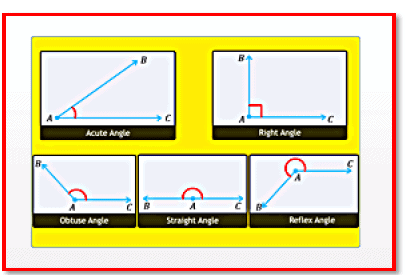-An angle is formed when lines or line segments meet.

-The lines that form an angle are called the sides or the arms of the angle.

-The common end point is called the vertex of the angle.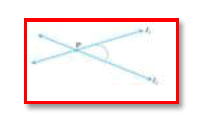-The angle formed by two lines or line segments can be an acute angle, where the measure of the angle is less than 90°.

-The angle formed by two lines or line segments can be a right angle, where the measure of the angle is90°.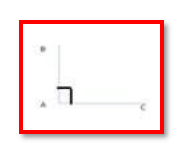-The angle formed by two lines or line segments can be an obtuse angle, where the measure of the angle is greater than 90°.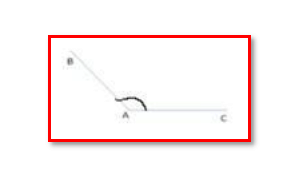-The angle formed by two lines or line segments can be a straight angle, where the measure of the angle is equal to 180°.

-The angle formed by two lines or line segments can be a reflex angle, where the measure of the angle is greater than 180°.

-When the sum of the measures of two angles is 90°, the angles are called complementary angles.

-When the sum of the measures of two angles is 180°, the angles are called supplementary angles.

-Adjacent angles have a common vertex and a common arm, and the non-common arms are on either side of the common arm.

-A linear pair is pair of adjacent angles whose non-common sides are opposite rays.

-Vertically opposite angles are opposite to each other and are equal.

-Adjacent angles have no common interior points.

-The angles in a linear pair are supplementary.

-Angles may have a common vertex and a common arm.

-Two angles are said to be adjacent angles if they have a common side or arm.

-Two adjacent angles can be either supplementary or complementary.

-If two adjacent angles are supplementary, then they form a straight angle, and are also called a linear pair.

-Two angles that are not adjacent but have a common vertex are called vertically opposite angles.

-Vertically opposite angles are equal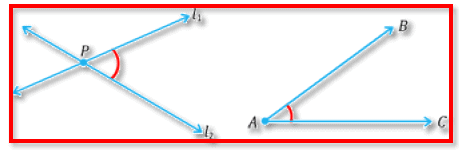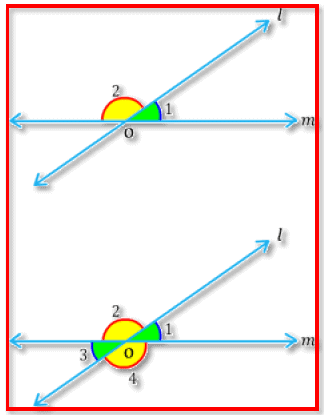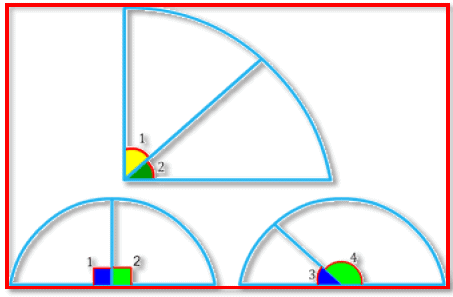Pairs of Lines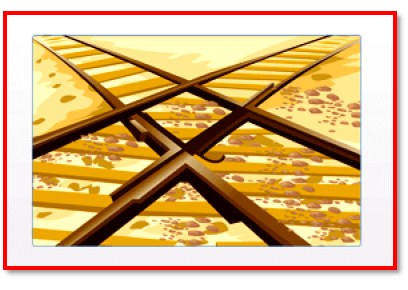-Lines that meet at a point are called intersecting lines.

-Lines that meet at a point are called intersecting lines.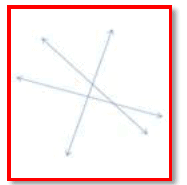-Lines that always remain the same distance apart and never meet are called parallel lines.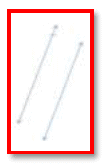-A line that intersects two or more lines at distinct points is called a transversal.

-When two lines are intersected by a transversal, pairs of corresponding angles, alternate angles and interior angles are formed.

-Angles formed on the same side of a transversal, on the same side of the two lines and at corresponding vertices are called corresponding angles.

-Angles formed on the opposite sides of the transversal at the two distinct points of intersection and between the two lines are called alternate interior angles.

-Angles formed on the opposite sides of the transversal at the two distinct points of intersection but outside the two lines are called alternate exterior angles.

-Angles that have different vertices, lie on the same side of the transversal and are interior angles are called consecutive interior angles or allied or co-interior angles.

-Each pair of interior angles on the same side of the transversal are supplementary, each pair of corresponding angles are equal and each pair of alternate interior angles are equal. -If a transversal cuts two lines such that the pairs of corresponding angles are equal, then the lines are parallel.

-Eight angles are formed when a transversal intersects two lines.

-The angles that lie between the lines are called interior angles.

-The angles that lie on the outer sides of the lines are called exterior angles.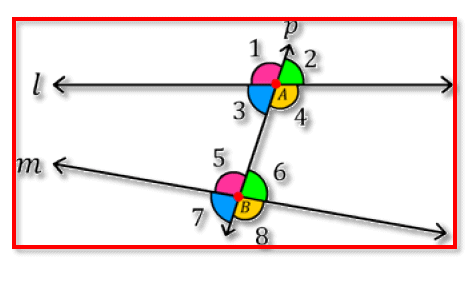The document Chapter Notes - Lines and Angles Notes | Study Mathematics (Maths) Class 7 - Class 7 is a part of the Class 7 Course Mathematics (Maths) Class 7.
All you need of Class 7 at this link: Class 7

## Mathematics (Maths) Class 7

168 videos|252 docs|45 tests
 Use Code STAYHOME200 and get INR 200 additional OFF

## Mathematics (Maths) Class 7

168 videos|252 docs|45 tests

Track your progress, build streaks, highlight & save important lessons and more!

,

,

,

,

,

,

,

,

,

,

,

,

,

,

,

,

,

,

,

,

,

;